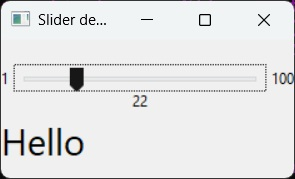# wxPython - Slider Class

A slider presents the user with a groove over which a handle can be moved. It is a classic widget to control a bounded value. Position of the handle on the groove is equivalent to an integer between the lower and upper bounds of the control.

wxPython API contains wx.Slider class. It offers same functionality as that of Scrollbar. Slider offers a convenient way to handle dragging the handle by slider specific wx.EVT_SLIDER event binder.

The definition of wx.Slider constructor takes the following eight parameters −

```wx.Slider(parent, id, value, minValue, maxValue, pos, size, style)
```

Slider’s lower and upper values are set by minValue and maxValue parameters. The starting value is defined by the value parameter.

Many style parameter values are defined. Following are some of them −

S.N. Parameters & Description
1

wxSL_HORIZONTAL

Horizontal slider

2

wxSL_VERTICAL

Vertical slider

3

wxSL_AUTOTICKS

Displays tickmarks on the slider

4

wxSL_LABELS

Displays the min, max, and current value

5

wxSL_MIN_MAX_LABELS

Displays the min and max value

6

wxSL_VALUE_LABEL

Displays the current value only

The useful methods of wx.Slider class are −

S.N. Methods & Description
1

GetMin()

Returns the minimum value of the slider

2

GetMax()

Returns the maximum value of the slider

3

GetValue()

Returns the current value of the slider

4

SetMin()

Sets the minimum value of the slider

5

SetMax()

Sets the maximum value of the slider

6

SetRange()

Sets the minimum and maximum slider values

7

SetValue()

Sets the current value programmatically

8

SetTick()

Displays the tick mark at the given position

9

SetTickFreq()

Sets the tick interval between the min and max values

As the slider behaves similar to a scroll bar, the scroll bar event binders can also be used along with it.

S.N. Events & Description
1

wx.EVT_SCROLL

Processes the scroll event

2

wx.EVT_SLIDER

When the slider position changes, either by moving the handle or programmatically

## Example

In the example that follows, the slider is used to control the size of a label. First of all, a slider object is placed in a vertical box sizer below which is a StaticText.

```self.sld = wx.Slider(pnl, value = 10, minValue = 1, maxValue = 100,
style = wx.SL_HORIZONTAL|wx.SL_LABELS)

self.txt = wx.StaticText(pnl, label = 'Hello',style = wx.ALIGN_CENTER)
```

Wx.EVT_SLIDER binder is associated with OnSliderScroll() handler.

```self.sld.Bind(wx.EVT_SLIDER, self.OnSliderScroll)
```

The handler itself is fetching slider’s current value and using it as font size for the label’s text.

```def OnSliderScroll(self, e):
obj = e.GetEventObject()
val = obj.GetValue()
font = self.GetFont()
font.SetPointSize(self.sld.GetValue())
self.txt.SetFont(font)
```

The complete code is as follows −

```import wx

class Mywin(wx.Frame):

def __init__(self, parent, title):
super(Mywin, self).__init__(parent, title = title,size = (250,150))
self.InitUI()

def InitUI(self):
pnl = wx.Panel(self)
vbox = wx.BoxSizer(wx.VERTICAL)

self.sld = wx.Slider(pnl, value = 10, minValue = 1, maxValue = 100,
style = wx.SL_HORIZONTAL|wx.SL_LABELS)

vbox.Add(self.sld,1,flag = wx.EXPAND | wx.ALIGN_CENTER_HORIZONTAL | wx.TOP, border = 20)
self.sld.Bind(wx.EVT_SLIDER, self.OnSliderScroll)
self.txt = wx.StaticText(pnl, label = 'Hello',style = wx.ALIGN_CENTER)

pnl.SetSizer(vbox)
self.Centre()
self.Show(True)

def OnSliderScroll(self, e):
obj = e.GetEventObject()
val = obj.GetValue()
font = self.GetFont()
font.SetPointSize(self.sld.GetValue())
self.txt.SetFont(font)

ex = wx.App()
Mywin(None,'Slider demo')
ex.MainLoop()
```

Run the code and try dragging the slider handle to see the label’s font size changing. The above code produces the following output −wxpython_major_classes.htm